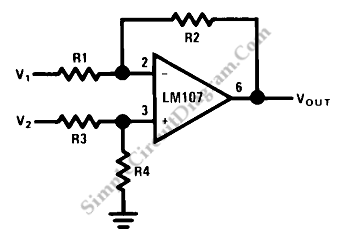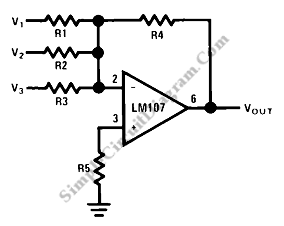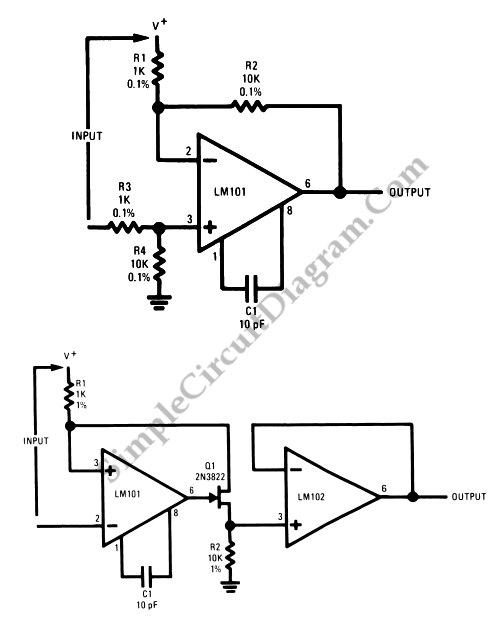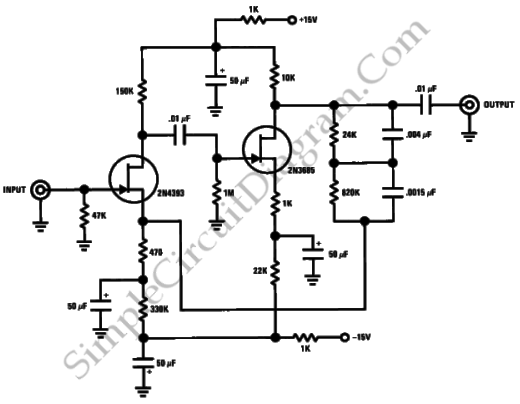Op-Amp Circuit: Difference AmplifierThis is a circuit of difference amplifier. This circuit is an inverting amplifier that allows the subtraction of two voltages and that is the summing amplifier’s complement.This circuit also allows the the cancellation of a signal common to the two inputs.This circuit can be used as a computational amplifier, in in rejecting a common mode signal or in making a […]

Op-Amp Circuit: Summing AmplifierThis is a summing amplifier circuit. This is an inverting circuit that provides an inverted output equal to the sum of all three input. This circuit has some advantages such as there is no interaction between the operation and inputs, and also it is very easily to implement the weighted averaging and summing on this circuit. Here is the circuit: […]

Op-Amp Application: Level Shifting AmplifierSometimes, in linear equipment design, it’s necessary to take a voltage which is referred to some dc level and generate an amplified output which is referred to ground. Using a differential amplifier similar to that shown in the circuit diagram below (the upper one) is the most straight-forward way of doing this. However, this circuit has a disadvantage, that is […]